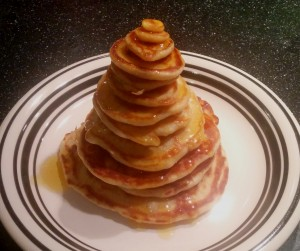## You're reading: Posts Tagged: open problems

### Hedetniemi’s Conjecture in graph theory disproved

In Quanta Erica Klarreich recently wrote up Yaroslav Shitov’s new counter example which disproves Stephen Hedetniemi’s 50 year old conjecture, original dissertation, that the number of colors required to color the tensor product of two graphs is the lesser of the numbers used to color the original graphs. These colorings have applications in areas from scheduling to seating plans, and it is clear from Klarreich’s reporting that mathematicians are excited about this result. In fact, Hedetniemi responded very positively when asked by Klarreich about the counter example, saying it “has a certain elegance, simplicity and definitive quality to it.” The counter-example may show Hedetniemi’s conjecture is not true, but Klarreich points out that we do not yet know just how false it is. So, while Shitov has closed one door on this problem, there are still many which are open.

### The 12th Polymath project has started: resolve Rota’s basis conjecture

Timothy Chow of MIT has proposed a new Polymath project: resolve Rota’s basis conjecture.

What’s that? It’s this:

… if $B_1$, $B_2$, $\ldots$, $B_n$ are $n$ bases of an $n$-dimensional vector space $V$ (not necessarily distinct or disjoint), then there exists an $n \times n$ grid of vectors ($v_{ij}$) such that

1. the $n$ vectors in row $i$ are the members of the $i$th basis $B_i$ (in some order), and

2. in each column of the matrix, the $n$ vectors in that column form a basis of $V$.

Easy to state, but apparently hard to prove!

### Open Season: Pancake Flipping

In this series of articles, I’m writing about mathematical questions we don’t know the answer to – which haven’t yet been proven or disproven. This edition is a topical one, for Pancake Day (Shrove Tuesday, celebrated in the UK this year on 9th February).Some of the best mathematical teasers are those which originate in a real-world problem – although the problem for pure mathematicians is that that happens much less often than it does for applied mathematicians, who are presented with interesting real-world problems all the time. That’s why it’s especially nice when a more pure one pops up, and that’s exactly what happened to mathematician Jacob E Goodman, back in 1975.

### Open Problems in Mathematics, a new open access journal

Here’s a nice idea: a journal for people to write about open problems, with the aim of inspiring someone to have a go at solving them. Open Problems in Mathematics is a new open-access journal set up by Krzysztof Burdzy and a few others, and it’s online now.

### Open Season: Prime Numbers (part 2)

In this short series of articles, I’m writing about mathematical questions we don’t know the answer to – which haven’t yet been proven or disproven. This is the third article in the series, and across two parts will discuss various open conjectures relating to prime numbers. This follows on from Open Season: Prime numbers (part 1).

So, we have a pretty good handle on how prime numbers are defined, how many of them there are, and how to check whether a number is prime. But what don’t we know? It turns out, quite a lot.

### Open Season: Prime Numbers (Part 1)

In this short series of articles, I’m writing about mathematical questions we don’t know the answer to – which haven’t yet been proven or disproven. This is the third article in the series, and across two parts will discuss various open conjectures relating to prime numbers.

I don’t think it’s too much of an overstatement to say that prime numbers are the building blocks of numbers. They’re the atoms of maths. They are the beginning of all number theory. I’ll stop there, before I turn into Marcus Du Sautoy, but I do think they’re pretty cool numbers. They crop up in a lot of places in maths, they’re used for all kinds of cool spy-type things including RSA encryption, and even cicadas have got in on the act (depending on who you believe).

### Open Season – The Perfect Cuboid

In this short series of articles, I’m writing about mathematical questions we don’t know the answer to – which haven’t yet been proven or disproven. This is the second article in the series, and considers a less well-known variant on an extremely well-known problem.

Ask anyone to name a theorem, and they’ll probably come up with one of the really famous ones, like Pythagoras’ theorem. This super-handy hypotenuse fact states that for a triangle with sides A, B and C, where the angle between A and B is a right angle, we have $C^2 = A^2 + B^2$. This leads us on to a nice bit of stamp-collecting – there are infinitely many triples of integers, A, B and C, which fit this equation, called Pythagorean Triples.

One well-known generalisation of this is to change the value $2$ to larger values, and go looking for triples satisfying $C^n = A^n + B^n$. But don’t – Andrew Wiles spent a good chunk of his life on proving that you can’t, for any value of $n>2$, find any such triples. The statement was originally made by Pierre De Fermat, and while Fermat famously didn’t write down a proof, it was the last of his mathematical statements to be gifted one – hence the name ‘Fermat’s Last Theorem’ – and proving it took over 350 years.